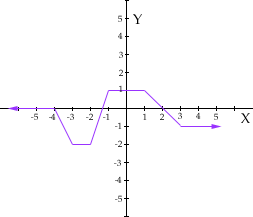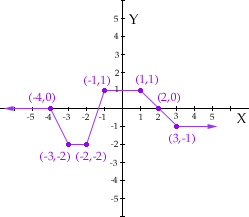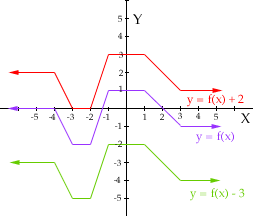Name: Monica

Who is asking: Other
Level: Secondary

Question:
Could you explain to me how one should go about graphing functions such as f(x), f(x+2), and so on. Also, how should you explain things such as constants and relationships among functions?

Hi Monica,

I have reproduced one of the graphs that you sent us. This is the graph of y = f(x).First I want to label the coordinates of some points on the graph.Since, for each point on the graph, the x and y coordinates are related by y = f(x), I can put the coordinates of these points in a list.

xy = f(x)
-40
-3-2
-2-2
-11
11
20
3-1

If now you wish to plot y = f(x) + 2 then, for each x value, use the table above to finf f(x) and then add 2 to get f(x) + 2. For example when x = -4 then f(x) = 0 so f(x) + 2 = 0 + 2 = 2 and x = -3 then f(x) = -2 so f(x) + 2 = -2 + 2 = 0. Thus I get

xy = f(x) y = f(x) + 2
-402
-3-20
-2-20
-113
113
202
3-11

On the graph below I plotted y = f(x) + 2 in red and also y = f(x) -3  in green.You also asked if f(x) + 2 and f(x + 2) are different. Yes they are. The placement of the parentheses makes a difference. For example with your graph above, when x = -4, f(x) = 0 and hence f(x) + 2 =0 + 2 = 2. But f(x + 2) = f(-4 + 2) = f(-2) = -2. In the second expression, since -4 + 2 is inside the parentheses you evaluate it first to get -2 and then use the table for y = f(x) to find f(-2).

Cheers,
Penny
Go to Math Central